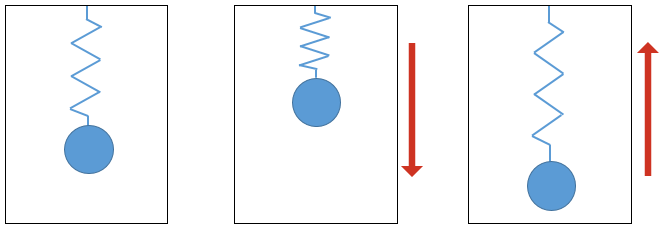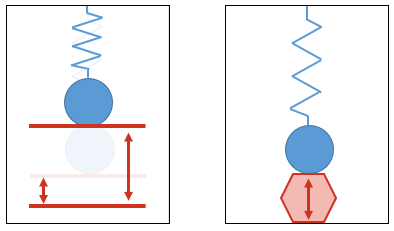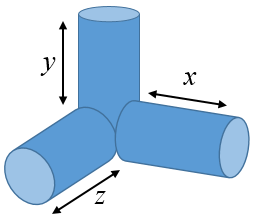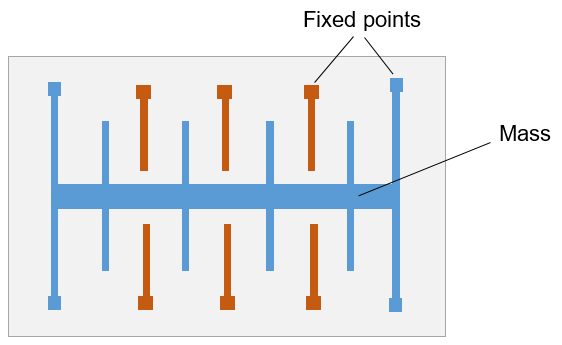# Accelerometers

The way an accelerometer works relies on the simple physics of Newton's laws of motion.

Newton's first law of motion states that a mass will remain in its current state of motion until acted on by an external force. So, if it is stationary it won't move unless it is pushed.

Newton's second law of motion - which can be summarised with the equation below - states that when a force is applied to a mass, the mass will accelerate in the same direction of the force.

$F = ma$

Without going any further into the physics, an accelerometer relies on the combined effect of these two laws to compare the motion of one mass with that of another mass which is initially stationary and which is tethered to the first in some flexible way. To illustrate, imagine the box shown in the figure below which contains a mass connected by a spring to its top surface. If an external force is applied in the downward direction, the suspended mass takes time to accelerate and the spring is compressed. If an upward force is applied, the spring stretches. As long as the characteristics of the mass and the spring are known, it is possible to use some clever maths to compare the motions of the box and the mass to determine the force applied in each case.To turn the mass-in-a-box arrangement into a sensor, we need a way of measuring the position of the mass. This can be done in a number of ways of which the most common are variable capacitance where the electrical properties of two plates change as they are moved closer together or further apart, or the piezoelectric effect where the voltage across certain substances changes when the substance is squeezed. These two possibilities are illustrated in the next figure. The internal implementation doesn't really matter as long as the reading that is produce reflects the magnitude of the force applied.This arrangement will measure accelerations in one dimension - ie parallel to the orientation of the spring. To measure movement in three dimensions, three similar devices must be combined at right angles to each other as shown below. Note that the x, y and z dimensions are defined relative to the screen in an Android device.An electronic accelerometer, such as the type in a mobile device, uses exactly the same principles described above, but on a much smaller physical scale. The figure below shows the structure of a Micro-Electro-Mechanical (MEMS) accelerometer. This type of device is packaged just like any other anonymous black electronics component measuring a few millimeters square.When the device moves, the central mass (in blue) flexes around its fixed points. When this happens the gaps between the spines on the mass and those on the housing (shown in orange) changes. This in turn causes a change in capacitance which can be measured.Accelerometers